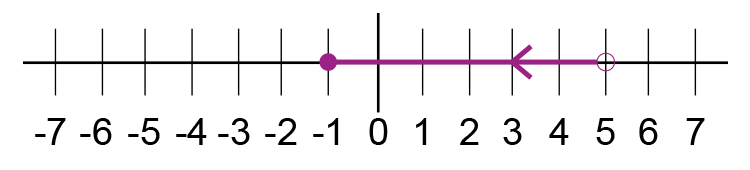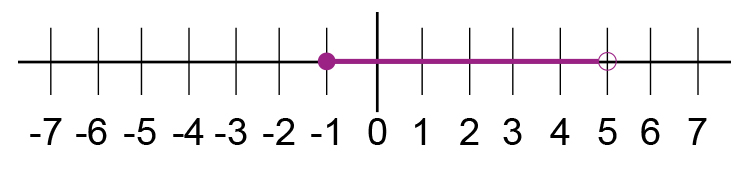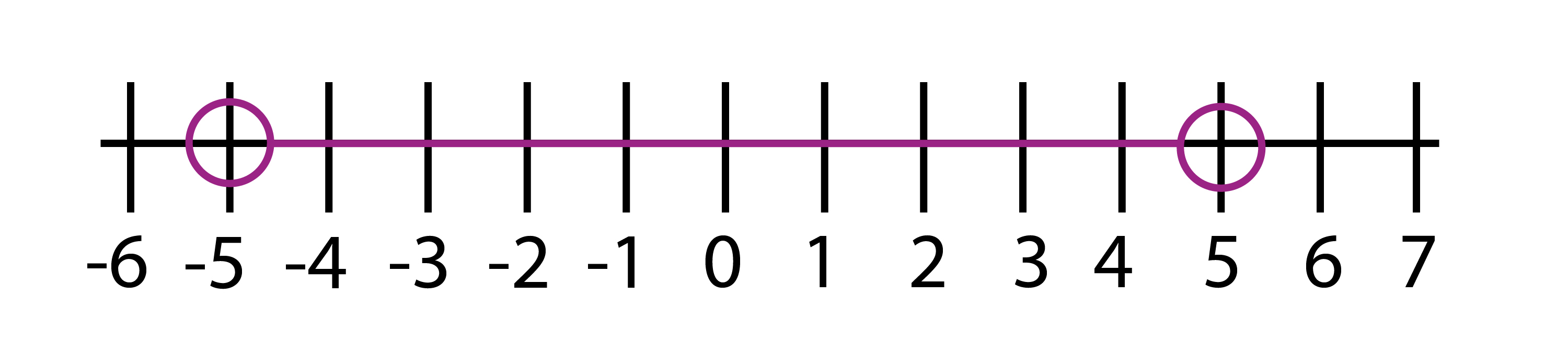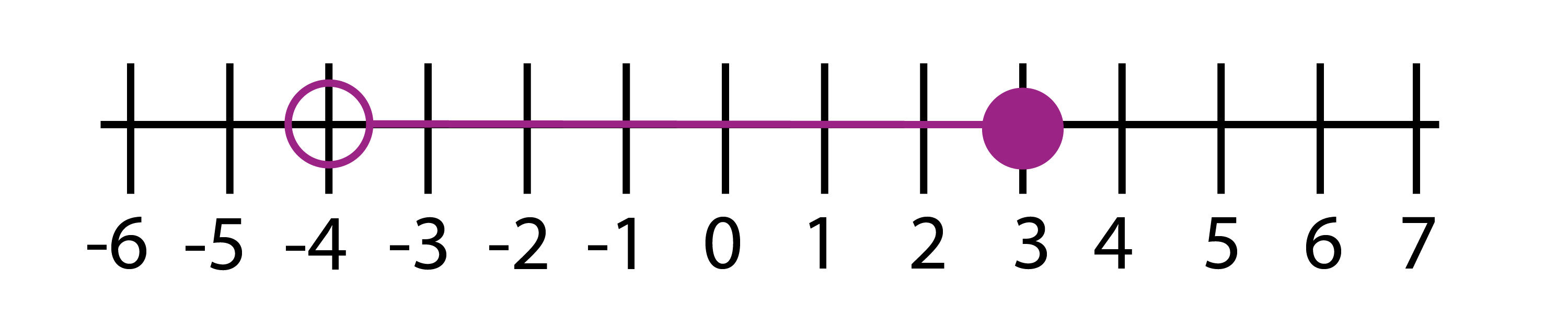# Three-part inequalities on a number line

For an expression like

-1<=x<5

Split this into two i.e.

-1<=x

And

x<5

-1<=x  this reads as -1  is less than or equal to x

x<5 this reads x is less than 5

These can be shown on a number line asOr as:i.e. without an arrow.

Example 1

Write down the inequality shown in the diagram below:Because x is the line between the two circles this can be read as:

The ring above -5  shows that -5  is less than x

(-5<\x)

The ring above +5  shows that x is less than 5

(x<\5)

Joining the two together

-5<\x<5

-5<\x<5

Example 2

Write down the inequality shown in the diagram below:Because x is the line between the two inequalities this can be read as

The ring above -4  shows that -4  is less than x

(-4<\x)

The circle above the 3  shows that x is less than or equal to 3

(x<=3)

Joining the two together

-4<\x<=3

-4<\x<=3